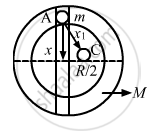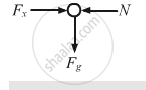Advertisement Remove all ads

# Assume that a Tunnel is Dug Along a Chord of the Earth, at a Perpendicular Distance R/2 from the Earth'S Centre Where R is the Radius of the Earth. - Physics

Sum

Assume that a tunnel is dug along a chord of the earth, at a perpendicular distance R/2 from the earth's centre where R is the radius of the earth. The wall of the tunnel is frictionless. (a) Find the gravitational force exerted by the earth on a particle of mass mplaced in the tunnel at a distance x from the centre of the tunnel. (b) Find the component of this force along the tunnel and perpendicular to the tunnel. (c) Find the normal force exerted by the wall on the particle. (d) Find the resultant force on the particle. (e) Show that the motion of the particle in the tunnel is simple harmonic and find the time period.

Advertisement Remove all ads

#### SolutionIf $\rho$  is the density of the earth, then mass of the earth $\left( M \right)$ is given by,

$M = \frac{4}{3}\pi R^3 \rho$

$\text {Similarly, mass} \left( M' \right) \text{ of the part of earth having radius } \left( x \right)\text{ is given by, }$ $M' = \frac{4}{3}\pi {x_1}^3 \rho$

$M' = \left( \frac{M}{R^3} \right) {x_1}^3$

(a) Let F be the gravitational force exerted by the earth on the particle of mass m. Then, its value is given by,

$F = \frac{GM'm}{x_1^2}$

$\text {Substituting the value of M' in the above equation, we get: }$

$F = \frac{GMm}{R^3}\frac{x_1^3}{x_1^2}$

$= \frac{GMm}{R^3} x_1 = \frac{GMm}{R^3}\sqrt{x^2 + \left( \frac{R^2}{4} \right)}$

(b)

$F_y = Fcos\theta$

$= \frac{GMm x_1}{R^3}\frac{x}{x_1} = \frac{GMmx}{R^3}$

$F_x = F\sin \theta$

$= \frac{GMm x_1}{R^3}\frac{R}{2 x_1} = \frac{GMm}{2 R^2}$

(c)$F_x = \frac{GMm}{R^2}$

$\because$ Normal force exerted by the wall N = Fx
(d)The resultant force is $\frac{GMmx}{R^3}$

(e) Acceleration = Driving force/mass

$= \frac{GMmx}{R^3 m}$

$= \frac{GMx}{R^3}$

$\Rightarrow$ a $\propto$  x      (the body executes S.H.M.)
$\frac{a}{x} = \omega^2 = \frac{GM}{R^3}$

$\Rightarrow \omega = \sqrt{\frac{Gm}{R^3}}$

$\Rightarrow T = 2\pi\sqrt{\frac{R^3}{GM}}$

Is there an error in this question or solution?
Advertisement Remove all ads

#### APPEARS IN

HC Verma Class 11, 12 Concepts of Physics 1
Chapter 12 Simple Harmonics Motion
Q 42 | Page 255
Advertisement Remove all ads

#### Video TutorialsVIEW ALL 

Advertisement Remove all ads
Share
Notifications

View all notifications

Forgot password?